last updated: 6th November 2021 - Day 215 - Deployable camera boom
How Much Water?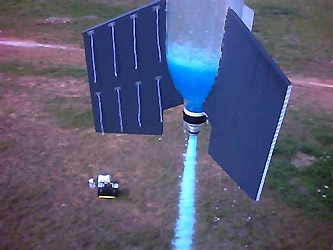How much water should I put in my water rocket?

Although this question seems simple enough, the answer depends on what you are trying to do with your rocket. This article looks at the answer in some detail from a theoretical as well as practical point of view.

General Rule of Thumb

If you just want to fly a rocket and don't care about the optimum amount of water, then a good rule of thumb to use is:

1/3 full of water, 2/3 full of air

If you are after a more accurate answer then read on ...

Why do we use water?

Newton's third law of motion tells us that throwing a mass in one direction causes us to move in the opposite direction. Water being heavy serves as a good mass to throw. This is also known as a 'reactive mass'. Water is inexpensive, available everywhere and is human friendly. Being a liquid it allows us to easily control the rate at which we throw it.

Why can't we fill up the whole rocket with water?

Water being essentially incompressible cannot store much energy when it is pressurised. Air on the other hand can store lots of energy when pressurised but is not very heavy and therefore doesn't work well as a reactive mass. In a water rocket the air provides the stored energy and the water provides the reactive mass. In order to achieve maximum performance a balance needs to be found between the amount of stored energy and the amount of reactive mass the rocket carries. Too much water and the rocket is too heavy, and not enough water means there isn't a lot of reactive mass to throw. Because the water and air share the same volume we have to trade one for the other.

Working Out the Optimum Amount

The optimum amount of water depends on a number of interdependent factors such as weight of the rocket, its drag coefficient, launch pressure, nozzle size etc. There is no simple rule to calculate the optimum amount of water for any particular rocket. Additionally you also need to take into consideration your desired goal for the rocket flight. You may be after the highest altitude, or carrying a heavy payload, or achieving the highest acceleration. These decisions will influence the optimum amount of water you use in your rocket.

So how can we work out the optimum?

Using Simulators

By far the easiest way to work out the optimal amount of water is to use one of the many water rocket simulators available on-line.

Procedure

The following procedure can be used with most simulators:

1. Enter your rocket and launcher parameters into the simulator and choose about 1/3 the capacity for the water fill. Most simulators have various extra parameters such as nozzle efficiency, launcher volume, air humidity, drag coefficient etc. that are needed to calculate the rocket performance. If you don't know what these are for your rocket then generally accepting the default values is close enough.

2. Run the simulation and the simulator will give you the predicted performance. Take a note of the performance result you are interested in.

3. Increase the water amount and re-run the simulation. The simulator will give you either higher or lower performance results.

4. If the simulator gives you lower performance then try to decrease the water amount and try again. If the simulator gives better performance then try increasing the water amount again.

5. By repeating these steps a number of times you can find the peak performance for a particular water fill amount.

NOTES:

• Some simulators [1,4] allow you to enter a range of values and the simulator will calculate the optimum amount for you.

• Most simulators, however, have limitations and will give less accurate results if you use extreme parameter values. Don't expect useful results if you are trying to simulate a 2000psi rocket or huge nozzles for example.

• Some of the better simulators are quite accurate at predicting flight performance, but if your rocket does not fit the typical profile, you can still work out the optimal water fill by flying your rocket number of times, varying the water amount and measuring your desired performance parameter.

Optimum Water Amounts

Now let's have a look at some of these rocket parameters and how they affect the optimum. Because there is a large variety of possible water rocket configurations we have chosen a pair of rockets that represent ones in common use:

 Rocket A Capacity 2 Liters Nozzle 9 or 22 mm Weight 150 or 300 grams Diameter 110 mm Drag Coefficient 0.3
 Rocket B Capacity 8 Liters Nozzle 9 or 22 mm Weight 700 or 1000 grams Diameter 90 mm Drag Coefficient 0.3

The following series of graphs describe the relationships between different rocket parameters and how they affect the optimum amount of water. Since we could optimize for different performance characteristics, we have chosen to optimize the amount of water for achieving the greatest altitude. For each point on the graph a simulation was run with the given parameters and we let the simulator calculate the optimum water amount.

Rocket Weight

The following graphs show how the optimum changes with respect to the rocket's dry weight.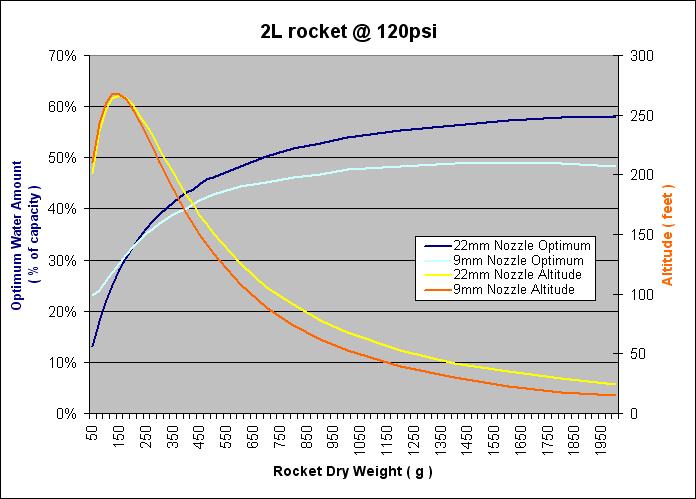Graph 1 - Optimum water amount vs. weight of rocket - Rocket A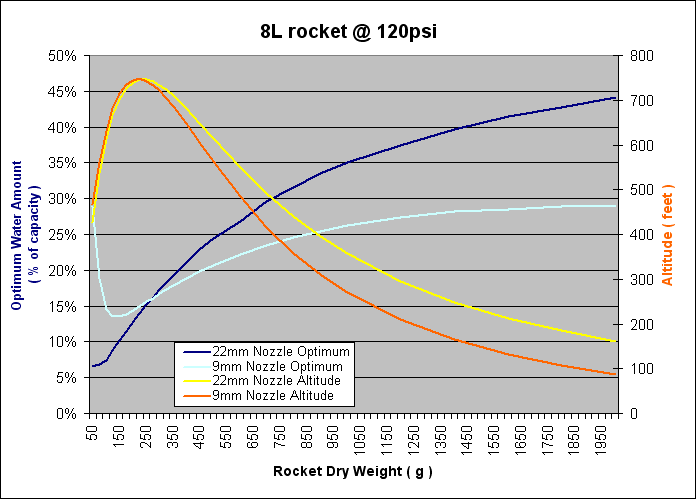Graph 2 - Optimum water amount vs. weight of rocket - Rocket B

You can see from Graph 1 that as expected the maximum altitude gained decreases as the dry weight of the rocket increases. However the optimum amount of water increases as well. At 2Kg the proportion of water is almost 58%. In graph 2 you can see that there is a big difference between the 22mm and 9mm nozzles in terms of the optimum water percentage. The graph also shows the optimum weight for rocket A is around 150 grams and about 175 grams for rocket B to achieve the greatest altitude.

Nozzle Size

The following graphs show how the optimum changes with respect to the nozzle size.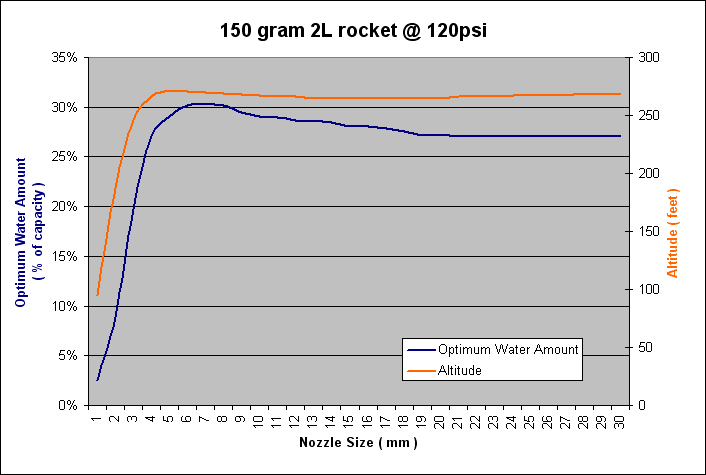Graph 3 - Optimum water amount vs. nozzle size - Rocket A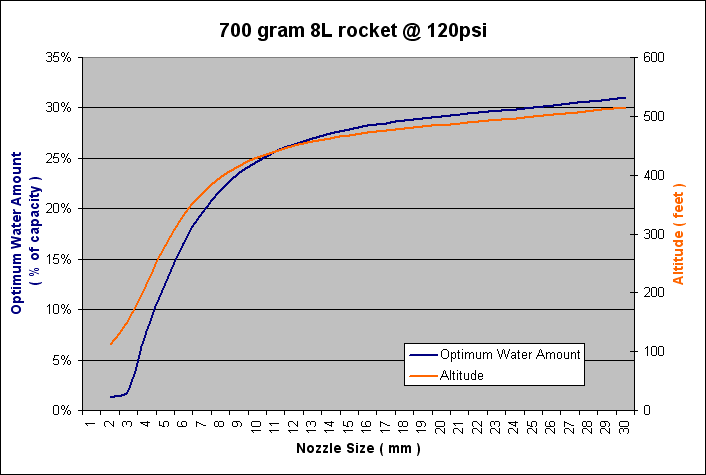Graph 4 - Optimum water amount vs. nozzle size - Rocket B

Graph 3 shows that for very small nozzles the optimum amount of water is quite low in proportion to the total volume of the rocket. This is expected because the thrust generated by a small nozzle isn't sufficient to lift a lot of weight. Notice also that for rocket A as you increase the nozzle size, the optimum amount of water slightly decreases for very little gain in altitude. Large nozzles mean higher acceleration but also higher drag. For rocket B though, you get higher performance as you increase the nozzle size and the optimum water amount also increases.

Pressure

The following graphs show how the optimum changes with respect to the launch pressure.Graph 5 - Optimum water amount of 150gram vs. pressure - Rocket A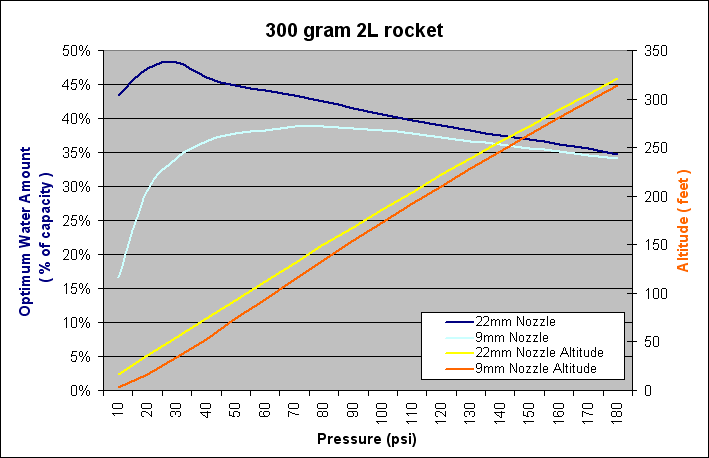Graph 6 - Optimum water amount of 300gram vs. pressure - Rocket A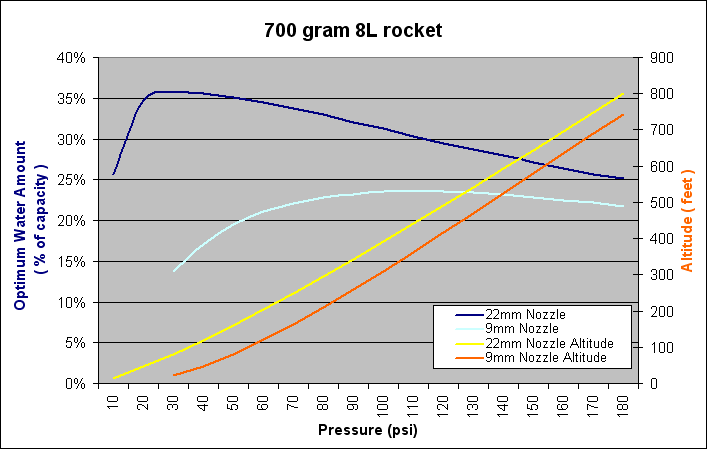Graph 7 - Optimum water amount of 700gram vs. pressure - Rocket B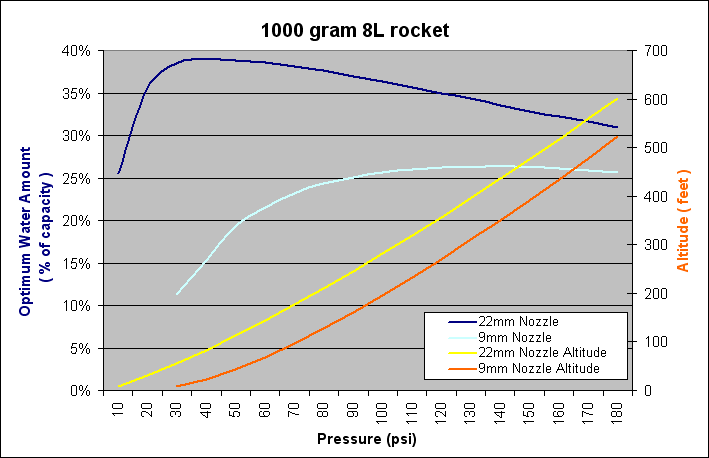Graph 8 - Optimum water amount of 1000gram vs. pressure - Rocket B

In the diagrams above, both common nozzles of 9mm and 22mm are shown. You can see that initially as pressure increases so does the optimum water amount, but then decreases again as the pressure increases. There is a large difference between the 22mm nozzle and 9mm nozzle. As the pressure increases the optimum amount of water peaks and the difference between 9mm and 22mm decreases.

Altitude

The next two graphs show the predicted altitude over a range of different water amounts at constant pressure.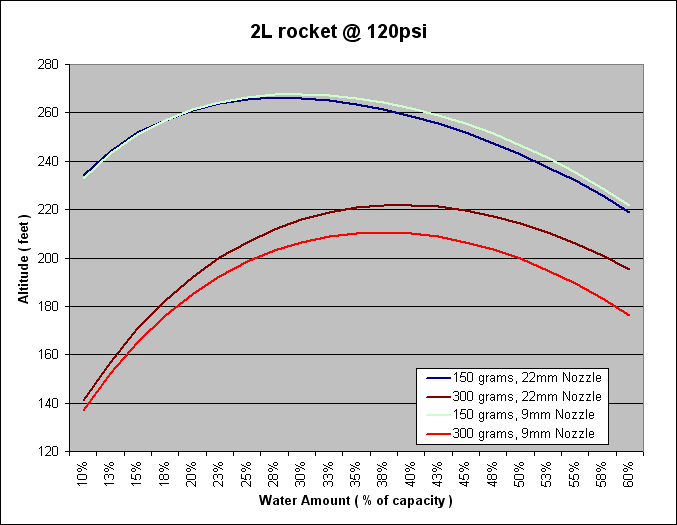Graph 9 - Predicted altitude vs. water amount - Rocket A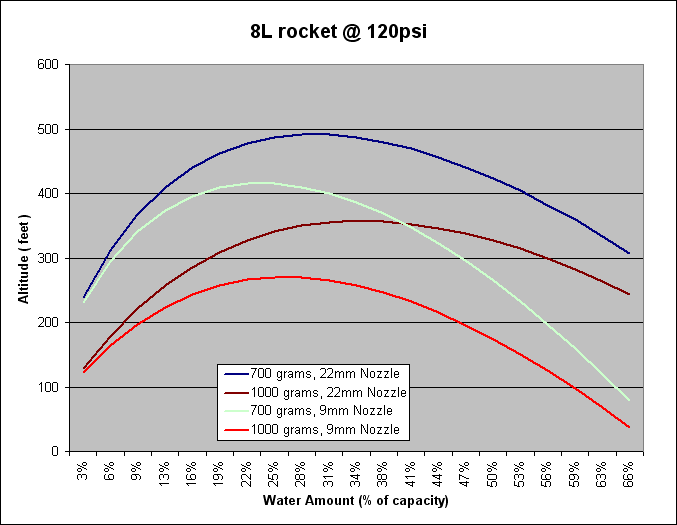Graph 10 - Predicted altitude vs. water amount - Rocket B

As you can see in graphs 9 and 10 the optimal amount of water peaks at different amounts depending on the the weight and size of nozzle. In graph 10 - while a heavy rocket with large nozzle peaked at 36% a lighter rocket with a smaller nozzle peaks at 23%. You can also see that the water amount is not critical near the peak altitude. In graph 9 for the 150 gram rocket you can see that water fill from 20%-40% results in only a small change in altitude of less than 10 feet or 4% of the overall altitude.

Practical Considerations

The following practical tips suggest why you may not necessarily want to use the optimum amount of water.

Stability

When you run a simulation to calculate the optimal amount of water you'll notice that there is quite a broad range of volumes of water around the optimum that give almost the same peak altitude (See Graphs 9 and 10). You may want to consider using less water than the optimum in your rocket that will result in higher acceleration and allow the rocket to reach a stable speed sooner.

This is particularly important if you are using reduced nozzles. Reduced nozzles have two drawbacks when it comes to rocket stability. They produce a lower take off thrust at the same pressure meaning that the rocket accelerates slower and hence reaches a stable speed later before the fins can actually start correcting the rocket's flight path. The other is the weight of the water. The heavy water near the tail can significantly move the center of gravity back on the rocket and cause the rocket to be unstable. With a reduced nozzle the water remains in the rocket longer and hence the center of gravity is further back for more of the flight. This means your rocket needs to use bigger fins and a longer guide rail compared to a higher accelerating rocket.

Pressure chamber restrictions

The construction of your rocket may also influence how much water you should use. If you build a large volume rocket out of bottles joined for example with Robinson couplings or tornado tubes, the optimal amount of water may exceed the capacity of the lowest bottle. Filling the rocket with water above the coupling will result in an effective rocket nozzle the size of the Robinson coupling while there is water still above the coupling. This may result in a loss of thrust on takeoff if the nozzle is larger than the lowest coupling.

In these situations only fill the bottom bottle with water allowing only air to pass through the coupling in order to restore some of the expected thrust on takeoff.

Can I add something to the water to make it heavier?

Yes you can, but it will typically result in lower expected altitude. Although the rocket will have higher thrust because it is expelling a heavier mass, it is also having to accelerate the heavier mass still in the rocket.

A water rocket actually benefits from a lower density liquid compared to water. The graphs below cover a range of liquid densities from alcohol (0.785g/cm3) to brine (1.23g/cm3) which are readily available. There are both lower and higher density liquids available, but these also tend to have other less desirable properties.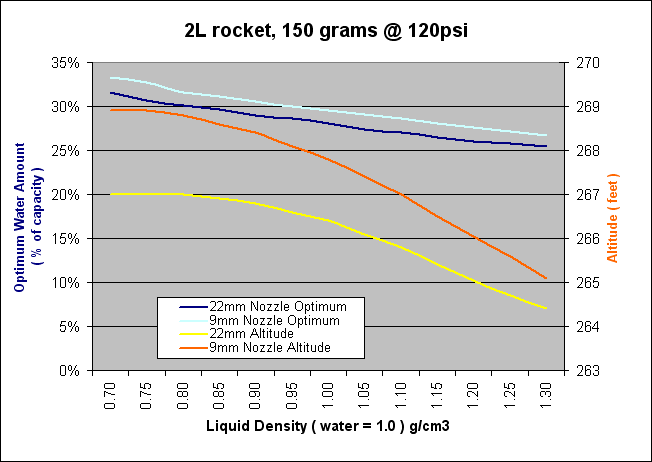Graph 9 - Predicted altitude vs liquid density - Rocket A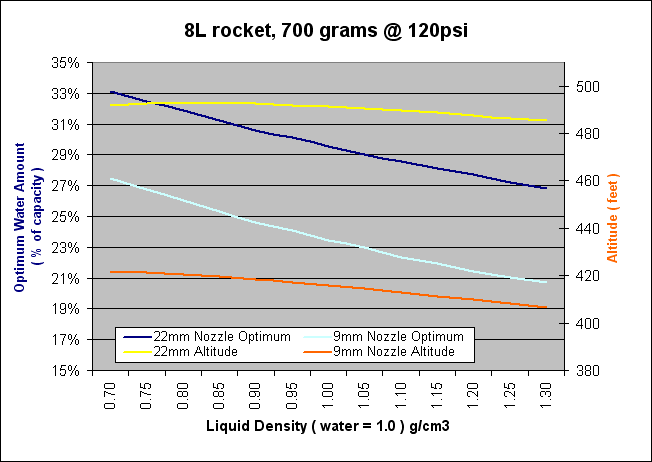Graph  - Predicted altitude vs liquid density - Rocket B

For each liquid density a simulator was used to work out the optimum amount of liquid.

Conclusion

As can be seen from the graphs above the optimum amount of water can greatly vary from 10%-60% depending on your rocket and launcher configuration, however, most rockets will not fall into these extremes. For larger nozzles the optimum is closer to 33% while for smaller nozzles the optimum is closer to 25%.

25th May 2011